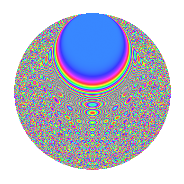# Properties

 Label 3009.2.iLevel 3009 Weight 2 Character orbit i Rep. character $$\chi_{3009}(353,\cdot)$$ Character field $$\Q(\zeta_{4})$$ Dimension 712 Sturm bound 720

# Related objects

## Defining parameters

 Level: $$N$$ = $$3009 = 3 \cdot 17 \cdot 59$$ Weight: $$k$$ = $$2$$ Character orbit: $$[\chi]$$ = 3009.i (of order $$4$$ and degree $$2$$) Character conductor: $$\operatorname{cond}(\chi)$$ = $$3009$$ Character field: $$\Q(i)$$ Sturm bound: $$720$$

## Dimensions

The following table gives the dimensions of various subspaces of $$M_{2}(3009, [\chi])$$.

Total New Old
Modular forms 728 728 0
Cusp forms 712 712 0
Eisenstein series 16 16 0

## Trace form

 $$712q - 720q^{4} - 8q^{7} + O(q^{10})$$ $$712q - 720q^{4} - 8q^{7} + 704q^{16} - 20q^{21} - 16q^{22} - 18q^{27} + 8q^{28} + 18q^{45} - 24q^{46} + 40q^{48} + 8q^{51} + 34q^{57} + 20q^{63} - 704q^{64} + 20q^{75} - 68q^{78} + 40q^{79} - 64q^{81} + 64q^{84} - 16q^{85} + 192q^{88} + O(q^{100})$$

## Decomposition of $$S_{2}^{\mathrm{new}}(3009, [\chi])$$ into irreducible Hecke orbits

The newforms in this space have not yet been added to the LMFDB.